### Home > MC2 > Chapter 1 > Lesson 1.3.1 > Problem1-122

1-122.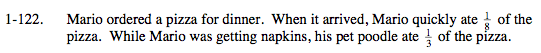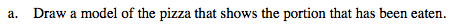There are various approaches to this question. You may numerically calculate the proportion of the pizza that has been eaten first, or divide the pizza into eighths and then into thirds to display the proportions through a picture.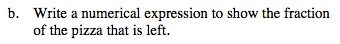Use a Giant One to represent the entire pizza.

Subtract that by the portions of the pizza that have been eaten to determine how much is left.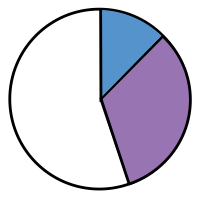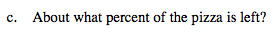Use a calculator to calculate the percent of pizza left.